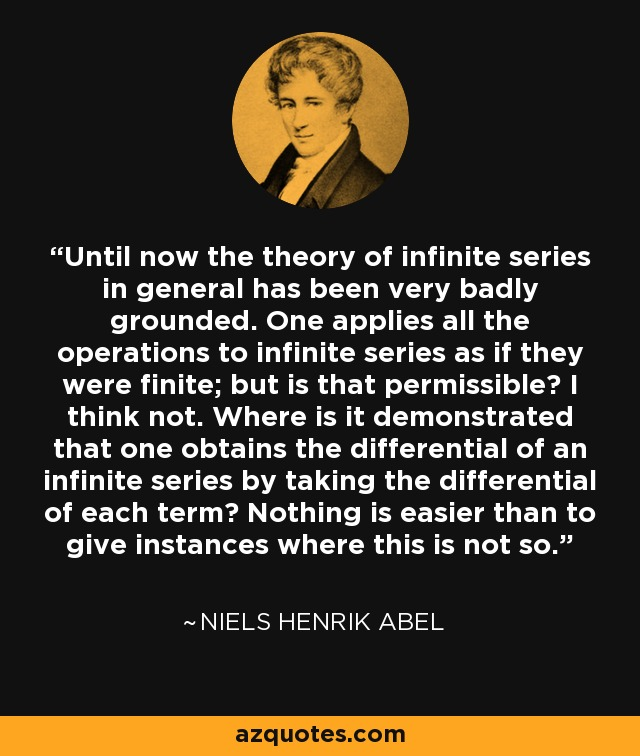Authors:
• Until now the theory of infinite series in general has been very badly grounded. One applies all the operations to infinite series as if they were finite; but is that permissible? I think not. Where is it demonstrated that one obtains the differential of an infinite series by taking the differential of each term? Nothing is easier than to give instances where this is not so.

### TopicsEmbed: